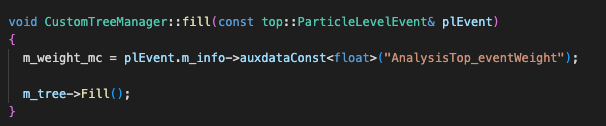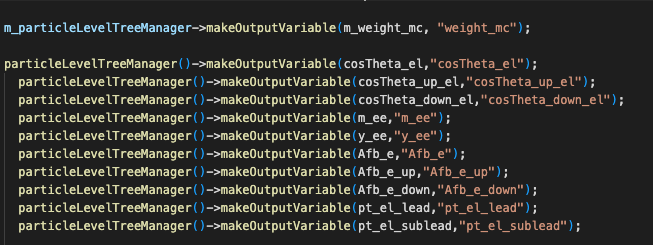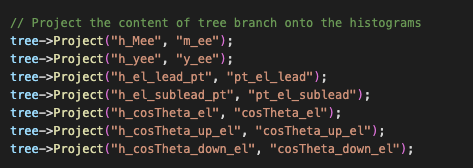# Applying weights to the branches of a TTree

Hi ROOT experts,

I am new to ROOT and I am looking at generating Drell-Yan MC samples. I have been using AnalysisTop to produce a tree where eahch branch contains my relevant variables for each event, which I want to fill into histograms. I want to apply a particular MC generator weight to each event entry when adding the variables into the tree. The trees are filled in the following code:Is there a way to apply the weight “m_weight_mc” to all the branches in the tree?

Note that as these weights are different depending on the event, I cannot perform a simple rescaling of the final histogram.

Thanks,
James

You can create a new (additional) branch with the weight.

How exactly would I do this?

You need to find the place where the “m_tree” is created (i.e., where all its branches are defined).

The branches of m_tree is defined as follows:

Would I need to multiply &obj by the weight?

You need to find the place where the “makeOutputVarible” is actually used.

I use MakeOutputVariable here:I don’t see how I can apply the weights here?

So, your tree already has a “`weight_mc`” branch (and the corresponding variable is set in the “`CustomTreeManager::fill`” method).
Just use it when filling histograms, e.g. → TH1::Fill ; TH2::Fill ; TH3::Fill

Yes, it does

I am currently filling the histograms using the “project” function:Can I apply the weight using project?

I am not sure how to loop over the branch entries and plot them into the histogram one-by-one, whilst weighting each entry by the corresponding weight.

`tree->Project("h_something", "something", "weight_mc");`

Thank you so much! I can’t believe it was that simple.